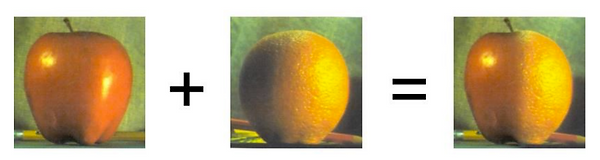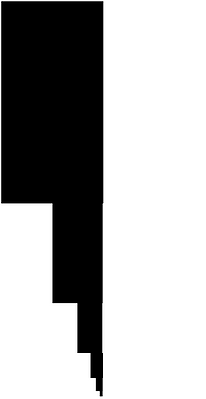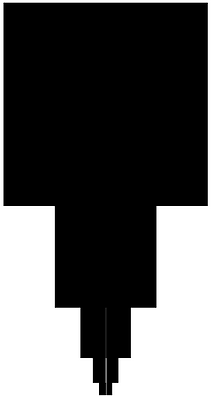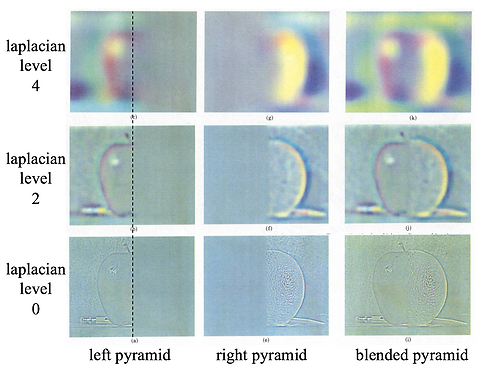top of page

# 2016

## Overview

The goal of this project is to seamlessly blend an object or texture from a source image into a target image. The simplest method would be to copy and paste pixels from one image directly to the other. Unfortunately, this will create noticeable seams, even if the backgrounds are similar. How can we get rid of these seams without doing too much perceptual damage to the source region? This project brings out a well-known blending algorithms in Python, the Laplacian pyramid blending.

Laplacian Pyramid Blending

Given a mask with black and white pixels only.

Given two input images, background image and foreground image.Background Image

Foreground Image

Result

# Concepts:

Quick Visual Concept on constructing a Laplacian Pyramid Blending Image:Resource from CSCI3290 Tutorial Notes

# Steps :

1. Input the three images, background image, foreground image and mask image.

2. Get pyramidN by using math function. pyramidN is used to determine how many times the image should be resized to make the pyramid. The number of total layers, PyramidN, depends on how large the image actually is since every images' width and height is the half of the former one. This implies that the larger the size is, the more layers there will be in the pyramid. We derive PyramidN as below:3. Create the pyramid of the three images by using the function "createPyramid" by passing the image and pyramidN into it. Code is as below:Noted that the number of layers of Gaussian Pyramid and Laplacian Pyramid is PyramidN-1, where that of Image Pyramid is PyramidN. In this piece of code, the for loop all run PyramidN times is only because of code implementation and utility.

Three different Pyramid lists of all three input images are as below:

# Foreground Image# Foreground Image# Background Image# Foreground Image4. Combine the laplacian pyramid of foreground and background by using the gaussian pyramid of the mask image. Formula:

LS(i,j) = GR(I,j,)*LA(I,j) + (1-GR(I,j))*LB(I,j)

L = Gaussian Pyramid of Mask * Laplacian Pyramid of Foreground

+ (1 - Gaussian Pyramid of Mask) * Laplacian Pyramid of Background

5. We get the smallest scale image. Resize it to the original image size, and it's the result!